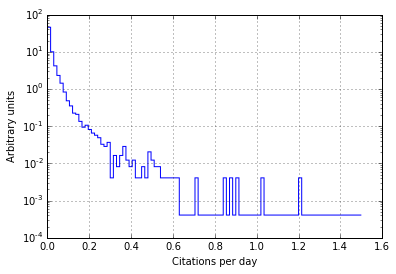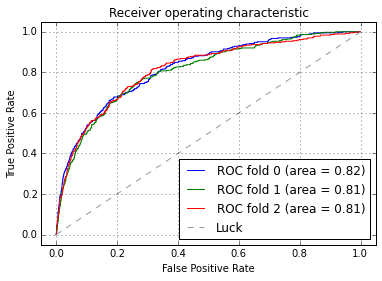# Getting Ahead on Interesting Papers

A large number of scientific articles are published on the arXiv every day. Most of them are not that interesting. This post is about how you can improve your chances of picking an article that is interesting by a factor of about three!

The dataset was collected for my previous post: Analysing the arXiv. One of the lessons learnt from looking at citation data for the articles I collected was that most of them are never cited. This supports the idea that most articles are not the interesting. Not even a fellow academic can be bothered to refer to it!

We will use NLP and Machine Learning to build a tool that can predict if an article will be interesting or not, at the moment it is published. This means we can not use information like how often it was cited to decide if the article is interesting.

So let's start this post about finding interesting things to read with some boring imports we will need later on:

In :
%matplotlib inline

In :
import datetime

import nltk
import numpy as np
import pandas as pd

import matplotlib.pyplot as plt

from scipy import interp

from sklearn import dummy
from sklearn import ensemble
from sklearn import grid_search
from sklearn import linear_model
from sklearn import cross_validation
from sklearn.pipeline import Pipeline, FeatureUnion
from sklearn.base import TransformerMixin, BaseEstimator, ClassifierMixin
from sklearn.metrics import roc_curve, auc
from sklearn.feature_extraction.text import CountVectorizer, TfidfTransformer, TfidfVectorizer


I am reusing data collected for my previous post, so head on over there to learn hwo to download it. Here I am just restoring a pandas dataframe from a HDF5 file:

In :
store = pd.HDFStore("/Users/thead/git/arxiv-experiments/hep-ex.h5")
#store['df'] = df
df = store['df']
store.close()


## What is interesting?¶

The most important question is: what is an interesting article? This is a highly contentious question, if you ask five people to give you a definition you will probably get six or seven. To make life simple we will define interesting as being in the top 10% of all the articles collected here.

Instead of predicting the total number of citations an article will receive over its lifetime. Instead we will use the number of citations a paper receives per day as a measure for interestingness.

An interesting article will be one that receives more than $f$ citations per day. There is some arbitrariness as to how to set $f$. We will set it so that 10% of articles have above $f$ citations per day.

Calculate the number of citations per day that an article receives and make a plot:

In :
end_of_2014 = datetime.date(2014,12,31)
df['cites_per_day'] = (df.citation_count /
((end_of_2014 - df.created).astype(int) / 1000000000 / (3600*24)))

df.cites_per_day.hist(bins=100, range=(0,1.5),
normed=True, log=True, histtype='step')
plt.xlabel("Citations per day")
plt.ylabel("Arbitrary units")

Out:
<matplotlib.text.Text at 0x112a54990>You can see this is a steeply falling distribution, even on a plot with the y axis in log-scale! To pick the cut off value we query the data frame for the 90% quantile and use that as a threshold.

In :
threshold = df.cites_per_day.quantile(0.9)
df['Y'] = df.cites_per_day>threshold

In :
df.Y.value_counts(normalize=True)

Out:
False    0.900006
True     0.099994
dtype: float64

As you can see, roughly 10% of entries are labelled True for interesting and the other 90% are labelled as False.

## The test-train divide¶

Next we define our pipeline for processing articles and feeding them to a classification algorithm. Beyond splitting the data set into a development and evaluation set, we also initialise some standard sklearn transformers to take a bunch of text and turn it into features for the classifier.

The ColumnExtractor is a handy class for extracting a specific column from a pandas data frame. By using it we can feed a data frame into our pipeline and have different columns extracted from it along the way. We use it to extract the abstract of every article and the title, and then process them separately.

In [ ]:
class ColumnExtractor(BaseEstimator, TransformerMixin):
def __init__(self, column=0):
"""Extract a column from a pandas.DataFrame

Use this transformer at the beginning of a
pipeline to extract the column of interest
from a data frame.
"""
self.column = column

def fit_transform(self, X, y=None, **kwargs):
self.fit(X, y, **kwargs)
return self.transform(X)

def transform(self, X, **kwargs):
return X[:,self.column]

def fit(self, X, y=None, **kwargs):
return self

X_dev, X_eval, y_dev, y_eval = cross_validation.train_test_split(df,
df.Y,
test_size=0.33,
random_state=78534)


## Feature Extraction¶

The next question is what to use as features for our classifier. We want to make a prediction as soon as an article is published. This limits us to features which are available from the beginning: title, abstract, authors, full text, etc. I suspect that the citations of an article would be a good feature to use to deduce its interestingness, but unfortunately this information is only available after a few weeks or months when people have started citing the article.

A standard approach for text classification is to tokenise the text and then transform it into a high dimensional vector. Each dimension of that vector represents one of the tokens in the input text. A quick demonstration on a bit of nonsense text:

In :
train = ["hello world world tiger bunny hopping",
"tiger world"]
test = ["hello world world tiger tiger",
"bunny hopping camel"]
c = CountVectorizer()
# learn this vocabulary, with 5 unique tokens
# the vectorizer will produce a 5D vector when
# transforming text
c.fit(train)
# this is the mapping of token to dimension
print c.vocabulary_
# tokenise new text and display the 5D vector
c.transform(test).toarray()

{u'tiger': 3, u'world': 4, u'hello': 1, u'hopping': 2, u'bunny': 0}

Out:
array([[0, 1, 0, 2, 2],
[1, 0, 1, 0, 0]])

You can see how both bits of text are transformed into 5D vectors. Something to keep in mind: Words which were not part of the training set are simply dropped.

The vectorizer by itself is not enough though, using the raw counts of each token has several problems including:

• it does not take into account the overall length of a document
• uninformative words like "the" or "and" which appear in every document receive a very high count

Both of these are addressed by the second step of the pipeline which turns the raw counts into a "term-frequency times inverse document-frequency" vector. Term-frequencey is the count of a token divided by the total number of tokens in a document. Inverse document-frequency scales down tokens found in a large number of documents, thereby giving them less weight.

In :
t = TfidfTransformer()
t.fit(c.transform(train))
print t.transform(c.transform(test)).toarray()

[[ 0.          0.44499628  0.          0.63323704  0.63323704]
[ 0.70710678  0.          0.70710678  0.          0.        ]]


ss

## Feature Pipeline¶

Let's assemble our complete pipeline. Both the title and abstract pipelines use bi-grams as tokens isntead of simple words, as well as a modified token_pattern which does not match numbers, only words.

In :
# pretty names for the dataframe column indices
TITLE = 0
ABSTRACT = 1

# sub-pipeline for extracting the abstract
vect = CountVectorizer(stop_words='english',
token_pattern=u'(?u)\\b[A-z][A-z]+\\b')
abstract_pipe = Pipeline([('abstracts', ColumnExtractor(ABSTRACT)),
('vect', vect),
('tfidf', TfidfTransformer())
])

# sub-pipeline for extracting the title
title_pipe = Pipeline([('title', ColumnExtractor(TITLE)),
('vect', CountVectorizer(stop_words='english',
token_pattern=u'(?u)\\b[A-z][A-z]+\\b')),
('tfidf', TfidfTransformer())
])
# combined pipeline
text_clf = Pipeline([('features', FeatureUnion([('abstract', abstract_pipe),
('title', title_pipe)])),
('clf', linear_model.SGDClassifier())
# use DummyClassifier to convince yourself we do better
# than pure luck
#('clf', dummy.DummyClassifier())
])


## Baseline¶

Running the pipeline with all hyperparameters set to their default values establishes a baseline:

In :
scores = cross_validation.cross_val_score(text_clf, X_dev, y_dev,
cv=3, scoring='roc_auc')
scores

Out:
array([ 0.79032066,  0.77259801,  0.76336835])

Using the area under the ROC curve as performance metric we get to around 0.78 or so.

By grid searching the hyperparameter space we can improve this a bit. This takes quite a bit of time to run, so skip ahead a few cells to get the parameter set which I found to perform best.

In :
parameters = {
'features__title__vect__max_df': (0.25, 0.5, 0.75, 1.0), #1.0 is best it seems
#'features__title__vect__min_df': (0.0, 0.25, 0.5, 0.75), #0.0 seems best
##'vect__max_features': (None, 5000, 10000, 50000), #no limit wins
#'features__abstract__vect__ngram_range': ((1, 1), (1, 2)),  # unigrams or bigrams, bigrams win
'features__title__vect__ngram_range': ((1, 1), (1, 2)),  # unigrams or bigrams,
##'tfidf__use_idf': (True, False),
##'tfidf__norm': ('l1', 'l2'),
#'clf__loss': ('hinge', 'log',), #log wins
#'clf__alpha': (1e-4, 1e-5, 1e-6, 1e-7), #alpha 1e-5
##'clf__penalty': ('l1', 'l2', 'elasticnet'), #l2
##'clf__n_iter': (10, 50, 80),
}

In [ ]:
grid = grid_search.GridSearchCV(text_clf, parameters,
n_jobs=-1, #verbose=1,
scoring='roc_auc')
grid.fit(X_dev, y_dev)

print("Best score: %0.3f"%grid.best_score_)
print("Best parameters set:")
best_parameters = grid.best_estimator_.get_params()
for param_name in sorted(parameters.keys()):
print("\t%s: %r"%(param_name, best_parameters[param_name]))


## Grid Search Results¶

This is the best set of parameters I found by grid searching the hyperparameter space. To get an unbiased estimated of the performance we evaluate it on the so far unseen "evaluation" set. Investing a few hours of computing time to find these parameters was only marginally successful as the performance is only slightly better than the default set.

In :
best_parameters = {'clf__alpha': 1e-05,
'clf__class_weight': 'auto',
'clf__penalty': 'l2',
'clf__loss': 'log',
'features__abstract__vect__ngram_range': (1, 2),
'features__title__vect__ngram_range': (1, 2),
'features__title__vect__max_df': 0.25,
}

text_clf.set_params(**best_parameters)
scores = cross_validation.cross_val_score(text_clf, X_eval, y_eval,
cv=3, scoring='roc_auc')
scores

Out:
array([ 0.79639601,  0.76458273,  0.82185392])

A more detailed way to look at the performance is to plot the ROC curve directly:

In :
cv = cross_validation.StratifiedKFold(y_dev, n_folds=3)

for i, (train, test) in enumerate(cv):
probas_ = text_clf.fit(X_dev[train], y_dev[train]).predict_proba(X_dev[test])
# Compute ROC curve and area under the curve
fpr, tpr, thresholds = roc_curve(y_dev[test], probas_[:, 1])
roc_auc = auc(fpr, tpr)
plt.plot(fpr, tpr, lw=1, label='ROC fold %d (area = %0.2f)'%(i, roc_auc))

plt.plot([0, 1], [0, 1], '--', color=(0.6, 0.6, 0.6), label='Luck')
plt.xlim([-0.05, 1.05])
plt.ylim([-0.05, 1.05])
plt.xlabel('False Positive Rate')
plt.ylabel('True Positive Rate')
plt.legend(loc="lower right")
plt.grid()
plt.show()## Nice!¶

We built a simple pipeline which can tell the difference between an interesting and a boring article posted to the arXiv using nothing more than the title and the abstract.

One thing to keep in mind though is that the number of boring articles is much, much larger than the number of interesting ones. This means if you tuned the decision threshold to have a false positive rate of 0.2 and a true-positive rate of 0.7 you would still get many more uninteresting articles than interesting ones. This goes by the name of Base rate fallacy.

If you receive 100 articles, then around 90 of them will be boring and the other ten will be interesting. Applying our classifier to these 100 articles it will classify about 25 as interesting, of which about seven will actually be interesting:

In :
fake = 90*0.2
real = 10*0.7
print "true positives: {} false positives: {}".format(real, fake)
print "Chance of reading something interesting: {}".format(real/(fake+real))

true positives: 7.0 false positives: 18.0
Chance of reading something interesting: 0.28


This post started life as a ipython notebook, download it or view it online.